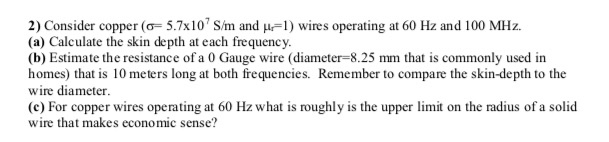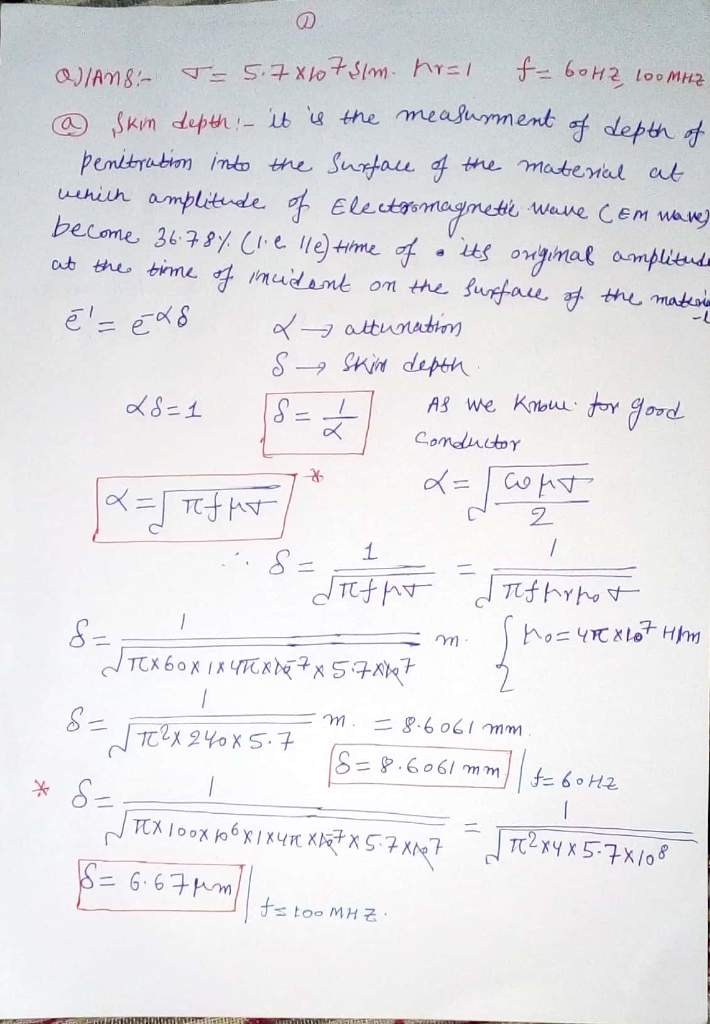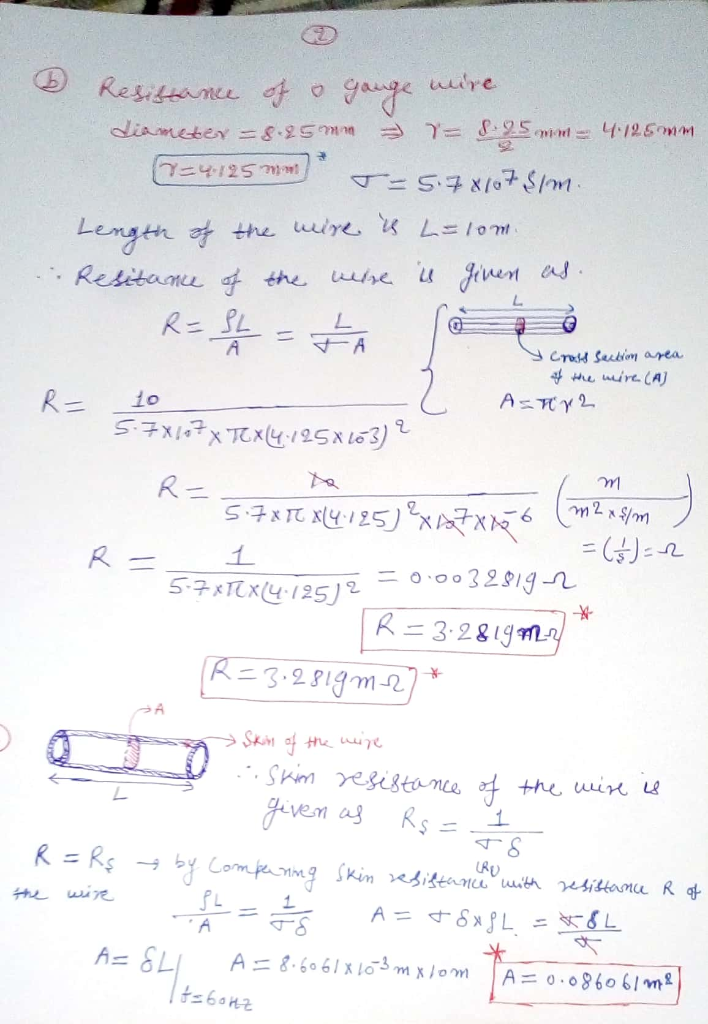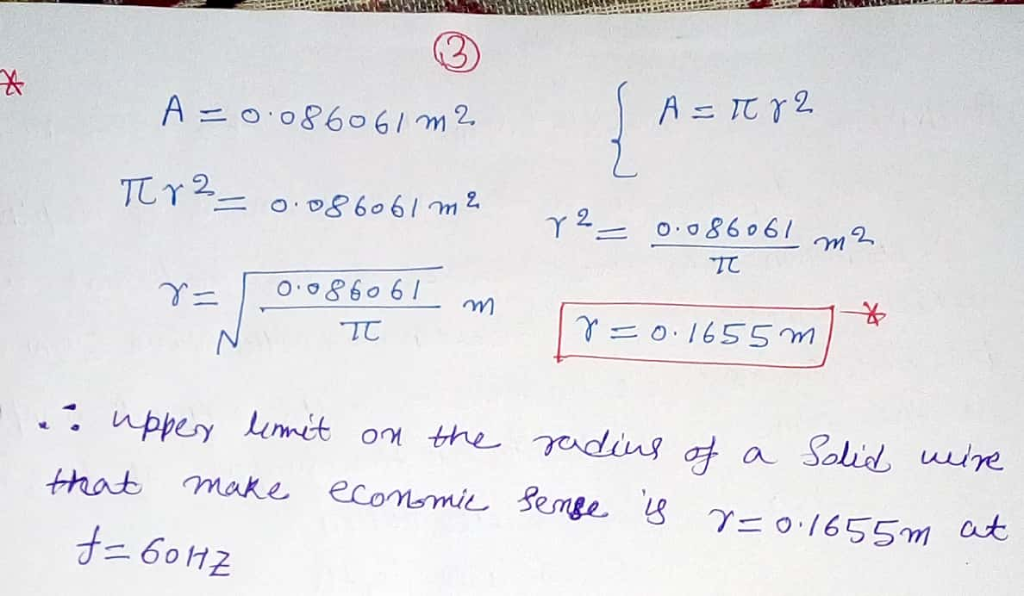# 2) Consider copper (o 5.7x10 S/m and u1) wires operating at 60 Hz and 100 MHz (a) Calculate the skin depth at each...2) Consider copper (o 5.7x10 S/m and u1) wires operating at 60 Hz and 100 MHz (a) Calculate the skin depth at each frequency (b) Estimate the resistance of a 0 Gauge wire (diameter-8.25 mm that is commonly used in homes) that is 10 meters long at both frequencies. Remember to compare the skin-depth to the wire diameter (c) For copper wires operating at 60 Hz what is roughly is the upper limit on the radius of a solid wire that makes econo mic sense?